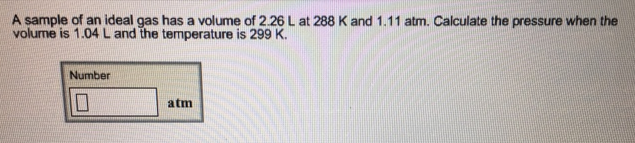# Problem: A sample of an ideal gas has a volume of 2.26 L at 288 K and 1.11 atm. Calculate the pressure when the volume is 1.04 L and the temperature is 299 K.

###### FREE Expert Solution
95% (84 ratings)###### Problem Details

A sample of an ideal gas has a volume of 2.26 L at 288 K and 1.11 atm. Calculate the pressure when the volume is 1.04 L and the temperature is 299 K.